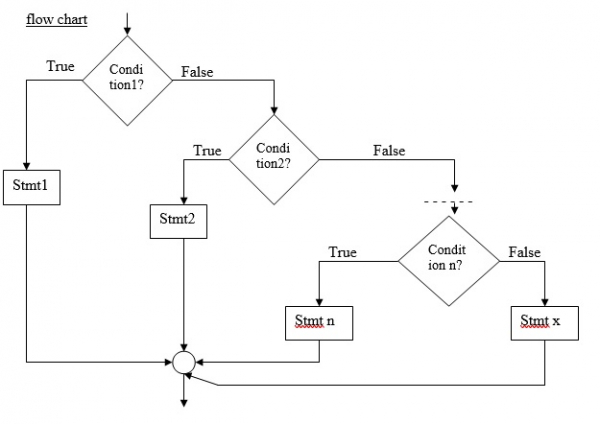# Explain else-if ladder statement in C language

CServer Side ProgrammingProgramming

This is the most general way of writing a multi-way decision.

## Syntax

Refer the syntax given below −

if (condition1)
stmt1;
else if (condition2)
stmt2;
- - - - -
- - - - -
else if (condition n)
stmtn;
else
stmt x;## Algorithm

Refer the algorithm given below −

START
Step 1: Declare int variables.
Step 2: Read a,b,c,d values at runtime
Step 3: i. if(a>b && a>c && a>d)
Print a is largest
ii.else if(b>c && b>a && b>d)
Print b is largest
iii. else if(c>d && c>a && c>b)
Print c is largest
iv. else
print d is largest
STOP

## Example

Following is the C program to execute Else If Ladder conditional operators −

Live Demo

#include<stdio.h>
void main (){
int a,b,c,d;
printf("Enter the values of a,b,c,d: ");
scanf("%d%d%d%d",&a,&b,&c,&d);
if(a>b && a>c && a>d){
printf("%d is the largest",a);
}else if(b>c && b>a && b>d){
printf("%d is the largest",b);
}else if(c>d && c>a && c>b){
printf("%d is the largest",c);
}else{
printf("%d is the largest",d);
}
}

## Output

You will see the following output −

Run 1:Enter the values of a,b,c,d: 2 4 6 8
8 is the largest
Run 2: Enter the values of a,b,c,d: 23 12 56 23
56 is the largest

Consider another C program which display the grade of student using else if ladder −

Live Demo

#include<stdio.h>
int main(){
int marks;
printf("Enter the marks of a student:\n");
scanf("%d",&marks);
if(marks <=100 && marks >= 90)
else if(marks < 90 && marks>= 80)
else if(marks < 80 && marks >= 70)
else if(marks < 70 && marks >= 60)
else if(marks < 60 && marks > 50)
else if(marks == 50)
else if(marks < 50 && marks >= 0)
printf("Fail");
else
printf("Enter a valid score between 0 and 100");
return 0;
}

## Output

You will see the following output −

Run 1:
Enter the marks of a student:78
Grade=A